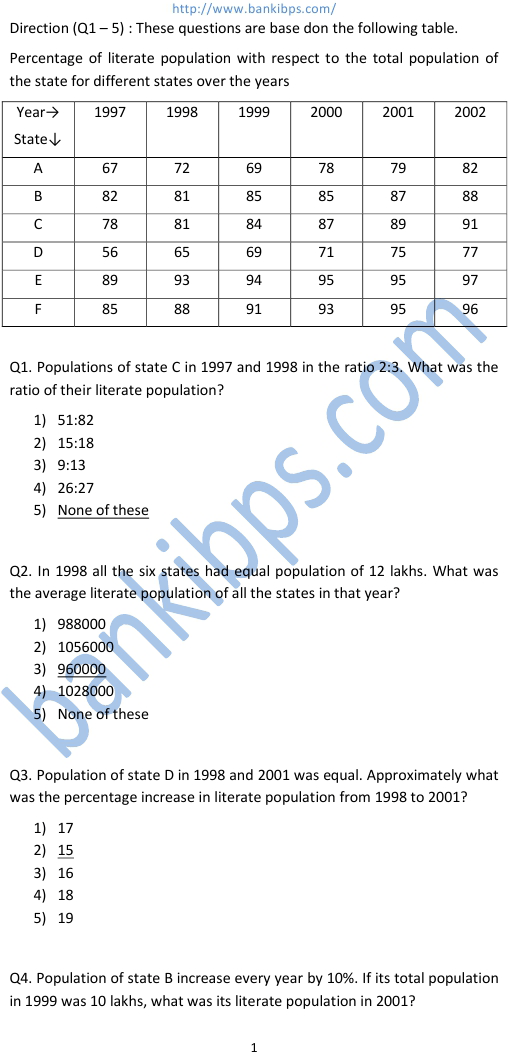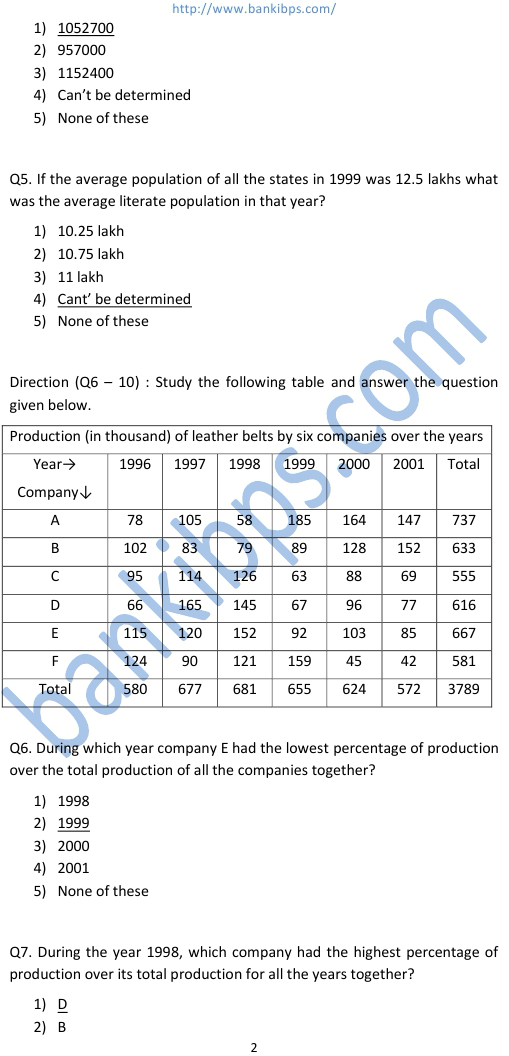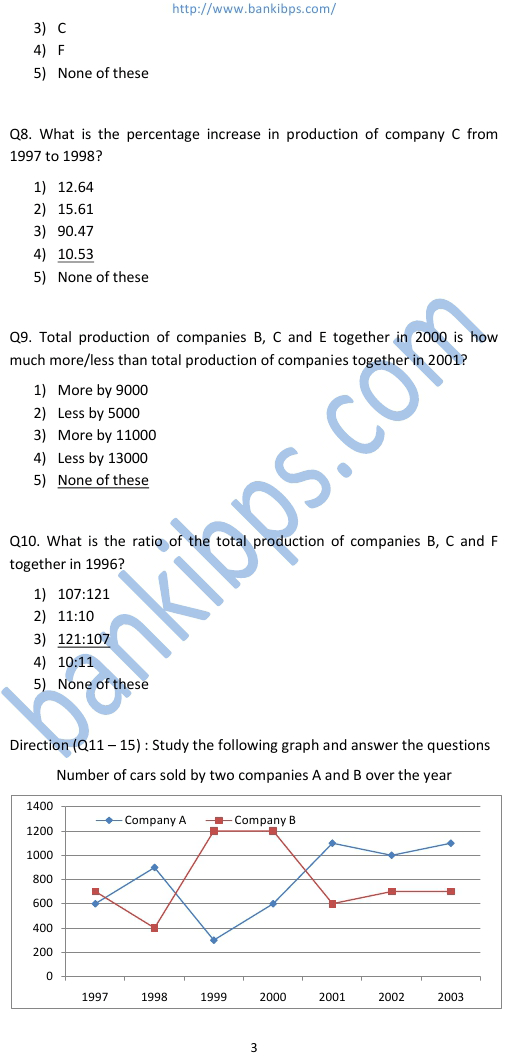# Data Analysis and Interpretation Solved Questions

Q1. Populations of state C in 1997 and 1998 in the ratio 2:3. What was the ratio of their literate population? data analysis and interpretation solved questions 1) 51:82 2) 15:18 3) 9:13 4) 26:27 5) None of these Q2. In 1998 all the six states had equal population of 12 lakhs. What was the average literate population of all the states in that year? 1) 988000 2) 1056000 3) 960000 4) 1028000 5) None of these Q3. Population of state D in 1998 and 2001 was equal. Approximately what was the percentage increase in literate population from 1998 to 2001? 1) 17 2) 15 3) 16 4) 18 5) 19
Practice Exercise - 256 [ Data Interpretation Questions ]## data analysis and interpretation solved questions

### data analysis and interpretation questions and answers

#### data analysis and interpretation solved questions pdf

##### test of data analysis interpretation
###### data interpretation questions with solutions
data analysis and interpretation questions and answers. data analysis and interpretation solved questions pdf. test of data analysis & interpretation. data interpretation solved questions. how to solve data interpretation questions. test of data analysis and interpretation. test of data analysis interpretation. how to solve data interpretation. data interpretation questions. data interpretation questions with solutions. data interpretation sample questions. data analysis and interpretation examples. data interpretation questions with answers. data interpretation test questions. data interpretation test papers.